# MCQs on Electromagnetic Fields

##### Page 19 of 20. Go to page 1 2 3 4 5 6 7 8 9 10 11 12 13 14 15 16 17 18 19 20
01․ Find the length of a conductor which is moving with a velocity 0.4m/s in a magnetic field of 8T, inducing an emf of 20V.
0.5m.
5.25m.
0.6m.
6.25m.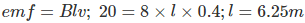02․ The magnetic field required to reduce the residual magnetism to zero is called
retentivity.
coercivity.
hysteresis.
saturation magnetism.

From the B-H curve, it is observed that the amount of magnetic field required in reverse direction to remove the residual magnetism is known as coercivity. It is given as the intercept on the negative H axis of the B-H curve.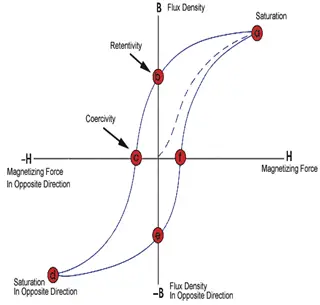03․ In order to minimize loss due to hysteresis the magnetic material should have
high hysteresis coefficient.
high resistivity.
large coercivity.
small B-H loop area.

Hysteresis loss is calculated as the area under B-H loop. So to minimize the hysteresis loss, B-H loop area should be made as small as possible.

04․ Find the strength of the magnetic field when a 2m long conductor is moving with a velocity 4m/s inducing an emf of 20V.
0.5T.
2.5T.
5T.
10T.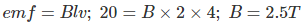05․ The shape of B-H curve for air gap is
parabola.
ellipse.
straight line.
hyperbola.

The shape of curve is straight line passing through origin as whatever amount of magnetic field is applied, air never saturates. So it will go on magnetizing. This straight line is also called air gap line.

06․ The coils having self inductance of 10 mH and 15 mH have an effective inductance of 40 mH, when connected in series aiding. What will be the equivalent inductance if we connect then in series opposing?
20 mH.
5 mH.
10 mH.
0 mH.

L = L1 + L2± 2M when ordinary, L = L1 + L2 + 2M. Hence, 40 = 10 + 15 + 2M ⇒ 2M = 15 mH. When opposing, L = L1 + L2 - 2M = 10 + 15 - 15 = 10 mH.

07․ Conductor is constant and field is varying then emf will be induced. This principle is called
virtually induced emf.
dynamically induced emf.
static induced emf.
none of the above.

According to the Faradays law of electromagnetic induction principle, emf will be induced whenever a conductor cuts a varying field or vice verse.

08․ Conductor is varying and field is fixed then emf will be induced. This principle is called
dynamically induced emf.
static induced emf.
both A and B.
none of the above.

According to the Faradays law of electromagnetic induction principle, emf will be induced whenever a conductor cuts a varying field or vice verse.

09․ For a linear electromagnetic circuit, which of the following statement is true?
Field energy is equal to the co-energy.
Field energy is greater than the co-energy.
Field energy is lesser than the co-energy.
Co-energy is zero.

The concept of co-energy and field energy can be shown from the following diagram as the variation of flux linkage with the current.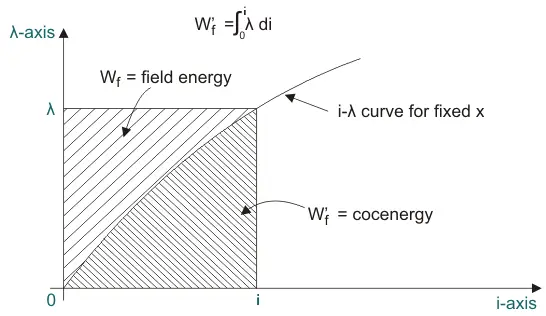For Linear electromagnetic circuit, the variation is a straight line and therefore the field energy and co-energy are equal.

10․ The developed electromagnetic force and or torque in electromechanical energy conversion system act in a direction that tends
to decrease the stored energy at constant mmf.
to decrease the co-energy at constant mmf.
to decrease the stored energy at constant flux.
to increase the stored energy at constant flux.

The expression for magnetic force is given as: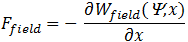This shows that stored energy of the field is reduced with the increase of x while flux linkages ψ are kept constant.

<<<17181920>>>